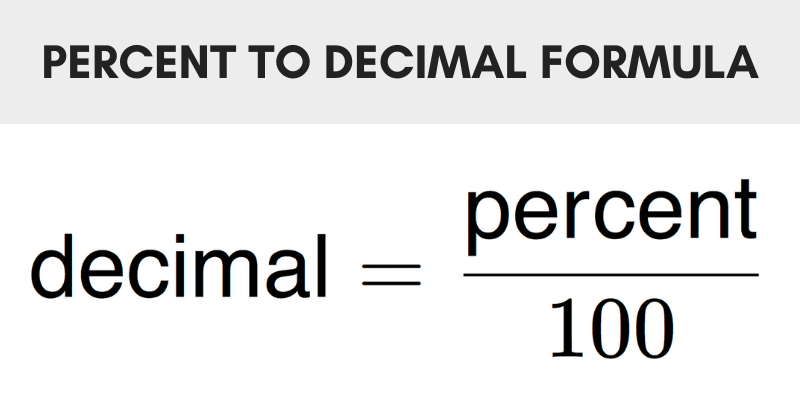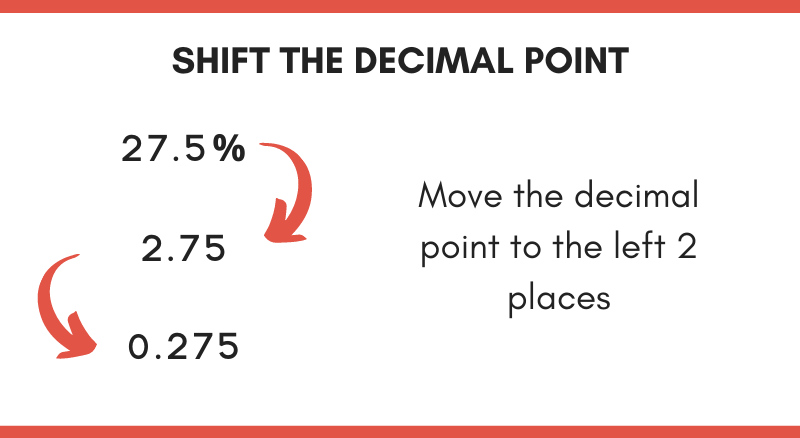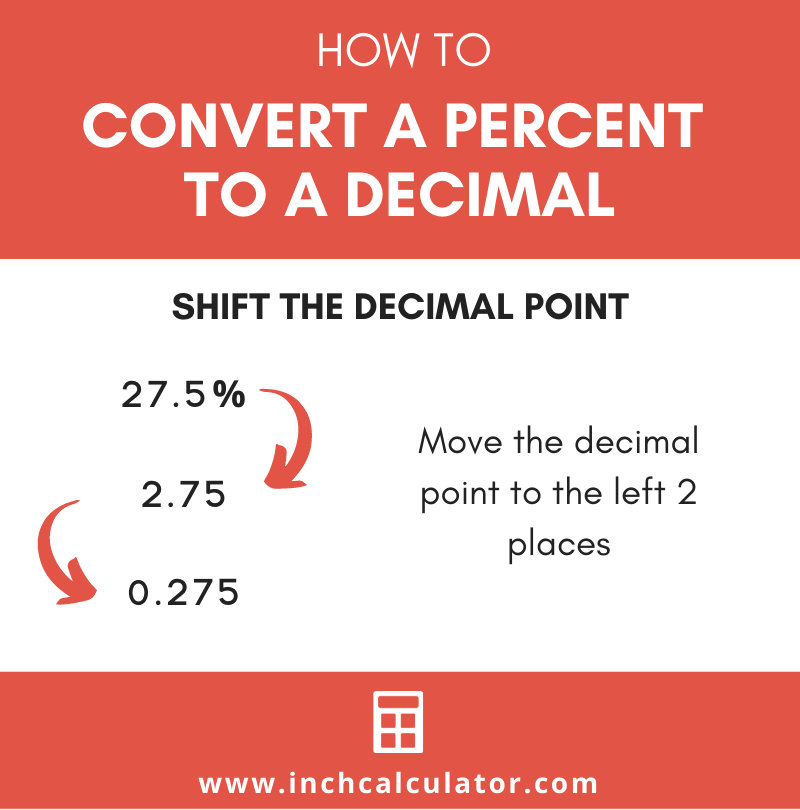# Percent to Decimal Calculator

Convert a percent to a decimal using the calculator below. Enter the percentage and submit to see the decimal result.

%

## Decimal:

0.5

### Steps to Convert Percentage to Decimal

decimal=percent / 100
Substitute the percentage in the equation
decimal=50 / 100
Solve
decimal=0.5
Learn how we calculated this below

## How to Convert From Percent to Decimal

Often a percent value needs to be converted to decimal form to allow it to be used in other equations. The decimal form does not have the % symbol.

Since percent literally means “per 100,” converting to decimal form is as simple as dividing the percentage by 100 and removing the % symbol.

There are two simple ways to convert a percent to decimal. The first is to use a formula, and the second is to shift the decimal point.

### Method One: Using the Percent to Decimal Formula

To convert from percent to decimal, use the following formula:

decimal = percent / 100

Thus, the decimal value is equal to the percent value divided by 100.

To convert a decimal back to a percent you can multiply the value by 100%.### Method Two: Shift the Decimal Point

The second method to convert a percent to decimal is simply moving the decimal point to the left two places and removing the percent symbol. For example:

27.5% = 0.275

Notice that in the example above, the decimal point was shifted to the left two places.Example: convert 48.5% to a decimal

decimal = percent / 100
decimal = 48.5 / 100
decimal = .485

See our complete set of percentage calculators for solving many types of problems.

## Percent to Decimal Conversion Chart

An alternative way to convert a percent to a decimal is to use our conversion table. The table below shows common percentages and their equivalent decimal values.

Percent to decimal conversion chart showing percentages and the equivalent decimal
Percent Decimal
0.1% 0.001
0.2% 0.002
0.3% 0.003
0.4% 0.004
0.5% 0.005
0.6% 0.006
0.7% 0.007
0.8% 0.008
0.9% 0.009
1% 0.01
1.5% 0.015
2% 0.02
2.5% 0.025
3% 0.03
3.5% 0.035
4% 0.04
4.5% 0.045
5% 0.05
5.5% 0.055
6% 0.06
6.5% 0.065
7% 0.07
7.5% 0.075
8% 0.08
8.5% 0.085
9% 0.09
9.5% 0.095
10% 0.1
15% 0.15
20% 0.2
25% 0.25
30% 0.3
35% 0.35
40% 0.4
45% 0.45
50% 0.5
55% 0.55
60% 0.6
65% 0.65
70% 0.7
75% 0.75
80% 0.8
85% 0.85
90% 0.9
95% 0.95
100% 1
200% 2
300% 3
400% 4
500% 5
600% 6
700% 7
800% 8
900% 9
1000% 10